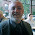### My infinity is bigger than your infinity!

Philosophy versus mathematics:

Mathematicians have taught us that, mathematically speaking, it is useful to talk about different types of infinity. This was a genuine accomplishment.

But whether or not some infinities are "larger" than other infinities is a philosophical question, turning on the concept of infinity itself, and not on any mathematical notions such as one-to-one correspondence.

### Comments

1.Countable, philosophic or mathematic?

1.That strikes me as a mathematical concept, but I have not given that much thought.

2.It's not a matter of philosophy that some infinities are larger than others. There are more real numbers between 0 and 1 than there are integers. I think some dude name Cantor proved this.

1.Amazing, rob! I am ADDRESSING Cantor. What Cantor showed was that the integers cannot be put in one-to-one correspondence with the reals. Yes, I UNDERSTAND that.

But that does not, necessarily, mean there are "more" reals than integers. Yes, in a FINITE set, it would prove that set A was bigger than set B. But what does it mean in terms of INFINITE sets?

Sorry, rob, that's a philosophical question.

2.What you have "proved" here, rob, is that you don't actually understand Cantor's mathematical finding!

3.Understanding what bigger means should not take a philosopher. If you can write an equation to prove something is that philosophy? Infinity is a common part of many mathematical equations.

4."Understanding what bigger means should not take a philosopher."

When thing A and thing B are both infinite sets?! Then in fact it DOES take a philosopher!

5.I think the Intuitionist school, Brouwer is the big name, would agree.

3.Gene, "2^Aleph_0 > Aleph_0" is a precise mathematical statement that can be proven in ZFC. Now you can say that the ">" symbol does not capture the philosophical concept of "greater than", but ">" still has a precise mathematical meaning.

1.That's fine, Keshav. I understand the mathematics perfectly well.